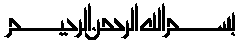Islamic Research Foundation International, Inc. Seeking Advancement of Knowledge through Spiritual and Intellectual Growth Articles 1 - 1000All Articles

# Interest Rate

This article or section needs copy editing for grammar, style, cohesion, tone or spelling.

Interest is a fee paid on borrowed capital. Assets lent include money, shares, consumer goods through hire purchase, major assets such as aircraft, and even entire factories in finance lease arrangements. The interest is calculated upon the value of the assets in the same manner as upon money. Interest can be thought of as “rent on money”.

The fee is compensation to the lender for foregoing other useful investments that could have been made with the loaned money. Instead of the lender using the assets directly, they are advanced to the borrower. The borrower then enjoys the benefit of the use of the assets ahead of the effort required to obtain them, while the lender enjoys the benefit of the fee paid by the borrower for the privilege. The amount lent, or the value of the assets lent, is called the principal. This principal value is held by the borrower on credit. Interest is therefore the price of credit, not the price of money as it is commonly - and mistakenly - believed to be. The percentage of the principal that is paid as a fee (the interest), over a certain period of time, is called the interest rate.

## The neutrality or factuality of this article or section may be compromised by weasel words, which can allow the implication of untrue information.

The charge of interest dates back to 1500 B.C.[citation needed] among the Sumerian and Egyptian cultures. References to the concept can be found in the religious text of the Abrahamic religions such as the counsel against excessive interest.

Interest is the price paid for the use of savings over a given period of time. In the Middle Ages, time was considered to be property of God. Therefore, to charge interest was considered to commerce with God’s property. Also, St. Thomas Aquinas, the leading theologian of the Catholic Church, argued that the charging of interest is wrong because it amounts to “double charging“, charging for both the thing and the use of the thing. The church regarded this as a sin of usury; nevertheless, this rule was never strictly obeyed and eroded gradually until it disappeared during the industrial revolution. Some scholars think that banking started among Jewish families because of the restrictions of the church.

… financial oppression of Jews tended to occur in areas where they were most disliked, and if Jews reacted by concentrating on moneylending to gentiles, the unpopularity - and so, of course, the pressure - would increase. This is that the Jews became an element in a vicious circle. The Christians, on the basis of the Biblical rulings, condemned interest-taking absolutely, and from 1179 those who practiced it were excommunicated. But the Christians also imposed the harshest financial burdens on the Jews. The Jews reacted by engaging in the one business where Christian laws actually discriminated in their favor, and so became identified with the hated trade of moneylending. 

Usury has always been viewed negatively by the Roman Catholic Church. The Second Lateran Council condemned any repayment of a debt with more money than was originally loaned, the Council of Vienna explicitly prohibited usury and declared any legislation tolerant of usury to be heretical, and the first scholastics reproved the charging of interest. In the medieval economy, loans were entirely a consequence of necessity (bad harvests, fire in a workplace) and, under those conditions, it was considered morally reproachable to charge interest.

In the Renaissance era, greater mobility of people facilitated an increase in commerce and the appearance of appropriate conditions for entrepreneurs to start new, lucrative businesses. Given that borrowed money was no longer strictly for consumption but for production as well, it could not be viewed in the same manner. The School of Salamanca elaborated various reasons that justified the charging of interest. The person who received a loan benefited and one could consider interest as a premium paid for the risk taken by the loaning party. There was also the question of opportunity cost, in that the loaning party lost other possibilities of utilizing the loaned money. Finally and perhaps most originally was the consideration of money itself as merchandise, and the use of one’s money as something for which one should receive a benefit in the form of interest.

Martín de Azpilcueta also considered the effect of time. Other things being equal, one would prefer to receive a given good now rather than in the future. This preference indicates greater value. Interest, under this theory, is the payment for the time the loaning individual is deprived of the money.

Economically, the interest rate is the cost of capital and is subject to the laws of supply and demand of the money supply. The first attempt to control interest rates through manipulation of the money supply was made by the French central Bank until 1847.

The first formal studies of interest rates and their impact on society were conducted by Adam Smith, Jeremy Bentham and Mirabeau during the birth of classic economic thought. In the early 20th century, Irving Fisher made a major breakthrough in the economic analysis of interest rates by distinguishing nominal interest from real interest. Several perspectives on the nature and impact of interest rates have arisen since then. Among academics, the more modern views of John Maynard Keynes and Milton Friedman are widely accepted.

Former Central President of the JUP Sahibzada Fazal Karim MNA has stated that the Council of Islamic ideology feels that Islamic banking ought to be interest-free by law.

## Types of interest

### Simple interest

Simple Interest is calculated only on the principal, or on that portion of the principal which remains unpaid.

The amount of simple interest is calculated according to the following formula: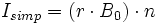where r is the period interest rate (10%=0.10), B0 the initial balance and n the number of time periods elapsed.

For example, imagine that a credit card holder has an outstanding balance of $2500 and that the interest rate is 12.99% per annum. If simple interest were charged on the balance the interest added at the end of 3 months would be,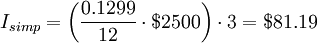and he would have to pay$2581.19 to pay off the balance at this time.

If instead he makes interest-only payments on the balance for 3 months at the same period rate the amount of interest paid would be,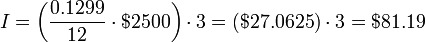The balance at the end of 3 months would still be $2500. So, interest-only payments result in amounts similar to simple interest but the difference is that the time value of money is not factored in and the steady payments have an additional cost which needs to be considered when comparing loans. To calculate the simple interest rate r one divides the interest paid by the number of periods and then divides the result by the initial balance. For example, given a$100 principal:

• Credit card debt where $1/day is charged: 1/100 = 1%/day. • Corporate bond where the first$3 are due after six months, and the second $3 are due at the year’s end: (3+3)/100 = 6%/year. • Certificate of deposit (GIC) where$6 is paid at the year’s end: 6/100 = 6%/year.

There are two complications involved in using the simple interest rate.

1. If you want to compare two different interest bearing offers and the time periods for each offer are different, a direct comparison is wrong because of the time value of money. For example paying $3 every six months costs more than$6 paid at year end. So the 6% bond cannot be ‘equated’ to the 6% GIC.
2. When interest is due, but not paid, the consequences are unclear. For example, does it remain ‘interest payable’, like the bond’s $3 payment after six months? Alternatively, will it be added to the original principal, as would typically be the case in the 1%/day borrowed via the credit card? In the latter case, it is no longer simple interest, but compound interest. ### Compound interest Main article: Compound interest In the short run, compound Interest is very similar to Simple Interest, however, as time goes on difference becomes considerably larger. The conceptual difference is that the principal changes with every time period, as any interest incurred over the period is added to the principal. Put another way, the lender is charging interest on the interest. Assuming that no part of the principal or subsequent interest has been paid, the amount of the debt, including principal and compound interest incurred, is calculated by the following formulas: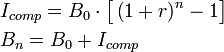where Icomp is the compound interest, B0 the initial balance, Bn the balance after n months and r the period rate. For example, if the credit card holder above chose not to make any payments the interest would accumulate,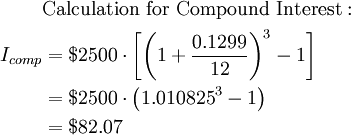So, at the end of 3 months the credit card holder’s balance would be$2582.07 and he would now have to pay $82.07 to get it down to the initial balance. Simple interest is approximately the same as compound interest for short periods of time. The interest payments indicate that more frequent payments is the better payment strategy. A problem with compound interest is that the resulting obligation can be difficult to interpret. To simplify this problem, a common convention in economics is to disclose the interest rate as though the term were one year, with annual compounding, yielding the effective interest rate. However, interest rates in lending are often quoted as nominal interest rates (i.e., compounding interest uncorrected for the frequency of compounding). The discussion at compound interest shows how to convert to and from the different measures of interest. Loans often include various non-interest charges and fees. One example are points on a mortgage loan in the United States. When such fees are present, lenders are regularly required to provide information on the ‘true’ cost of finance, often expressed as an annual percentage rate (APR). The APR attempts to express the total cost of a loan as an interest rate after including the additional fees and expenses, although details may vary by jurisdiction. In economics, continuous compounding is often used due to its particular mathematical properties. #### Fixed and floating rates Commercial loans generally use compound interest, but they may not always have a single interest rate over the life of the loan. Loans for which the interest rate does not change are referred to as fixed rate loans. Loans may also have a changeable rate over the life of the loan based on some reference rate (such as LIBOR and EURIBOR), usually plus (or minus) a fixed margin. These are known as floating rate, variable rate or adjustable rate loans. Combinations of fixed-rate and floating-rate loans are possible and frequently used. Less frequently, loans may have different interest rates applied over the life of the loan, where the changes to the interest rate are governed by specific criteria other than an underlying interest rate. An example would be a loan that uses specific periods of time to dictate specific changes in the rate, such as a rate of 5% in the first year, 6% in the second, and 7% in the third. ### Composition of interest rates In economics, interest is considered the price of money, therefore, it is also subject to distortions due to inflation. The nominal interest rate, which refers to the price before adjustment to inflation, is the one visible to the consumer (i.e., the interest tagged in a loan contract, credit card statement, etc). Nominal interest is composed by the real interest rate plus inflation, among other factors. A simple formula for the nominal interest is: i = r + π Where i is the nominal interest, r is the real interest and π is inflation. This formula attempts to measure the value of the interest in units of stable purchasing power. However, if this statement was true, it would imply at least two misconceptions. First, that all interest rates within an area that shares the same inflation (i.e. the same country) should be the same. Second, that the lender knows the inflation for the period of time that he/she is going to lend the money. One reason behind the difference between the interest that yields a Treasury bond and the interest that yields a Mortgage loan is the risk that the lender takes from lending money to an economic agent. In this particular case, the US government is more likely to pay than a private citizen. Therefore, the interest rate charged to a private citizen is larger than the rate charged to the US government. To take into account the information asymmetry aforementioned, both the value of inflation and the real price of money is changed to their expected values resulting in the following equation: it = rt + 1 + πt + 1 + σ Where it is the nominal interest at the time of the loan, rt + 1 is the real interest expected over the period of the loan, πt + 1 is the inflation expected over the period of the loan and σ is the representative value for the risk engaged in the operation. ### Cumulative interest or return Cumulative interest/return: This calculation is (FV/PV)-1. It ignores the ‘per year’ convention and assumes compounding at every payment date. It is usually used to compare two long term opportunities. Since the difference in rates gets magnified by time, so the speaker’s point is more clearly made. ### Other conventions and uses Other exceptions: • US and Canadian T-Bills (short term Government debt) have a different convention. Their interest is calculated as (100-P)/P where ‘P’ is the price paid. Instead of normalizing it to a year, the interest is prorated by the number of days ‘t’: (365/t)*100. (See also: Day count convention). The total calculation is ((100-P)/P)*((365/t)*100). This is equivalent to calculating the price by a process called discounting at a simple interest rate. • Corporate Bonds are most frequently payable twice yearly. The amount of interest paid is the simple interest disclosed divided by two (multiplied by the face value of debt). Flat Rate Loans and the Rule of 78s: Some consumer loans have been structured as flat rate loans, with the loan outstanding determined by allocating the total interest across the term of the loan by using the “Rule of 78s” or “Sum of digits” method. Seventy-eight is the sum of the numbers 1 through 12, inclusive. The practice enabled quick calculations of interest in the pre-computer days. In a loan with interest calculated per the Rule of 78s, the total interest over the life of the loan is calculated as either simple or compound interest and amounts to the same as either of the above methods. Payments remain constant over the life of the loan; however, payments are allocated to interest in progressively smaller amounts. In a one-year loan, in the first month, 12/78 of all interest owed over the life of the loan is due; in the second month, 11/78; progressing to the twelfth month where only 1/78 of all interest is due. The practical effect of the Rule of 78s is to make early pay-offs of term loans more expensive. For a one year loan, approximately 3/4 of all interest due is collected by the sixth month, and pay-off of the principal then will cause the effective interest rate to be much higher than the APY used to calculate the payments.  In 1992, the United States outlawed the use of “Rule of 78s” interest in connection with mortgage refinancing and other consumer loans over five years in term. Certain other jurisdictions have outlawed application of the Rule of 78s in certain types of loans, particularly consumer loans.  Certain other jurisdictions have outlawed application of the Rule of 78s in certain types of loans, particularly consumer loans.  Rule of 72: The “Rule of 72” is a “quick and dirty” method for finding out how fast money doubles for a given interest rate. For example, if you have an interest rate of 6%, it will take 72/6 or 12 years for your money to double, compounding at 6%. This is an approximation that starts to break down above 10%. ## Market interest rates There are markets for investments which include the money market, bond market, as well as retail financial institutions like banks, which set interest rates. Each specific debt takes into account the following factors in determining its interest rate: Opportunity cost: This encompasses any other use to which the money could be put, including lending to others, investing elsewhere, holding cash (for safety, for example), and simply spending the funds. Inflation: Since the lender is deferring his consumption, he will at a bare minimum, want to recover enough to pay the increased cost of goods due to inflation. Because future inflation is unknown, there are three tactics. • Charge X% interest ‘plus inflation’. Many governments issue ‘real-return’ or ‘inflation indexed’ bonds. The principal amount and the interest payments are continually increased by the rate of inflations. See the discussion at real interest rate. • Decide on the ‘expected’ inflation rate. This still leaves both parties exposed to the risk of ‘unexpected’ inflation. • Allow the interest rate to be periodically changed. While a ‘fixed interest rate’ remains the same throughout the life of the debt, ‘variable’ or ‘floating’ rates can be reset. There are derivative products that allow for hedging and swaps between the two. Default: There is always the risk the borrower will become bankrupt, abscond or otherwise default on the loan. The risk premium attempts to measure the integrity of the borrower, the risk of his enterprise succeeding and the security of any collateral pledged. For example, loans to developing countries have higher risk premiums than those to the US government due to the difference in creditworthiness. An operating line of credit to a business will have a higher rate than a mortgage. Creditworthiness of businesses is measured by bond rating services and individual’s credit scores by credit bureaus. The risks of an individual debt may have a large standard deviation of possibilities. The lender may want to cover his maximum risk. But lenders with portfolios of debt can lower the risk premium to cover just the most probable outcome. Deferred consumption: Charging interest equal only to inflation will leave the lender with the same purchasing power, but he would prefer his own consumption NOW rather than later. There will be an interest premium of the delay. See the discussion at time value of money. He may not want to consume, but instead would invest in another product. The possible return he could realize in competing investments will determine what interest he charges. Length of time: Time has two effects. • Shorter terms have less risk of default and inflation because the near future is easier to predict. Broadly speaking, if interest rates increase, then investment decreases due to the higher cost of borrowing (all else being equal). Interest rates are generally determined by the market, but government intervention - usually by a central bank- may strongly influence short-term interest rates, and is used as the main tool of monetary policy. The central bank offers to buy or sell money at the desired rate and, due to their control of certain tools (such as, in many countries, the ability to print money) they are able to influence overall market interest rates. Investment can change rapidly to changes in interest rates, affecting national income, and, through Okun’s Law, changes in output affect unemployment. ### Open market operations in the United States The effective federal funds rate charted over fifty years The Federal Reserve (often referred to as ‘The Fed’) implements monetary policy largely by targeting the federal funds rate. This is the rate that banks charge each other for overnight loans of federal funds. Federal funds are the reserves held by banks at the Fed. Open market operations are one tool within monetary policy implemented by the Federal Reserve to steer short-term interest rates. Using the power to buy and sell treasury securities, the Open Market Desk at the Federal Reserve Bank of New York can supply the market with dollars by purchasing T-notes, hence increasing the nation’s money supply. By increasing the money supply or Aggregate Supply of Funding (ASF), interest rates will fall due to the excess of dollars banks will end up with in their reserves. Excess reserves may be lent in the Fed funds market to other banks, thus driving down rates. ### Interest rates and credit risk It is increasingly recognized that the business cycle, interest rates and credit risk are tightly interrelated. The Jarrow-Turnbull model was the first model of credit risk which explicitly had random interest rates at its core. Lando (2004), Darrell Duffie and Singleton (2003), and van Deventer and Imai (2003) discuss interest rates when the issuer of the interest-bearing instrument can default. ### Money and inflation Loans, bonds, and shares have some of the characteristics of money and are included in the broad money supply. By setting i*n, the government institution can affect the markets to alter the total of loans, bonds and shares issued. Generally speaking, a higher real interest rate reduces the broad money supply. Through the quantity theory of money, increases in the money supply lead to inflation. This means that interest rates can affect inflation in the future. ## Interest in mathematics Jacob Bernoulli discovered the mathematical constant e by studying a question about compound interest. He realized that if an account that starts with$1.00 and pays 100% interest per year, at the end of the year, the value is $2.00; but if the interest is computed and added twice in the year, the$1 is multiplied by 1.5 twice, yielding $1.00×1.5² =$2.25. Compounding quarterly yields $1.00×1.254 =$2.4414…, and so on

Bernoulli noticed that this sequence can be modeled as follows:where n is the number of times the interest is to be compounded in a year.

## Formulas and Worksheets

The balance of a loan with regular monthly payments is augmented by the monthly interest charge and decreased by the payment so,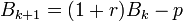.

where,

i = loan rate/100 = annual rate in decimal form (e.g. 10% = 0.10 The loan rate is the rate used to compute payments and balances.)

r = period rate = i/12 for monthly payments (customary usage for convenience)

B0 = initial balance (loan principal)

Bk = balance after k payments

k = balance index

p = period (monthly) payment

By repeated substitution one obtains expressions for Bk which are linearly proportional to B0 and p and use of the formula for the partial sum of a geometric series results in,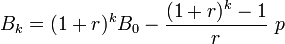A solution of this expression for p in terms of B0 and Bn reduces to,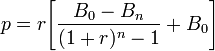To find the payment if the loan is to be paid off in n payments one sets Bn = 0.

The PMT function found in spreadsheet programs can be used to calculate the monthly payment of a loan: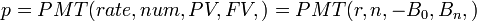An interest-only payment on the current balance would be,The total interest, IT, paid on the loan is,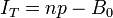The formulas for a regular savings program are similar but the payments are added to the balances instead of being subtracted and the formula for the payment is the negative of the one above. These formulas are only approximate since actual loan balances are affected by rounding. In order to avoid an underpayment at the end of the loan the payment needs to be rounded up to the next cent. The final payment would then be (1+r)Bn-1.

Consider a similar loan but with a new period equal to k periods of the problem above. If rk and pk are the new rate and payment, we now have,Comparing this with the expression for Bk above we note that,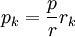The last equation allows us to define a constant which is the same for both problems,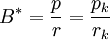and B_k can be written,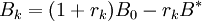Solving for rk we find a formula for rk involving known quantities and Bk, the balance after k periods,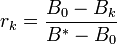Since B0 could be any balance in the loan, the formula works for any two balances separate by k periods and can be used to compute a value for the annual interest rate.

B* is a scale invariant since it doesn’t change with changes in the length of the period.

Rearranging the equation for B* one gets a transformation coefficient (scale factor),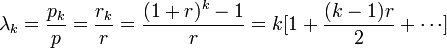(see binomial theorem)

and we see that r and p transform in the same manner,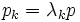The change in the balance transforms likewise,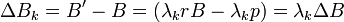which gives an insight into the meaning of some of the coefficients found in the formulas above. The annual rate, r12, assumes only one payment per year and is not an “effective” rate for monthly payments. With monthly payments the monthly interest is paid out of each payment and so should not be compounded and an annual rate of 12·r would make more sense. If one just made interest-only payments the amount paid for the year would be 12·r·B0.

Substituting pk = rk B* into the equation for the Bk we get,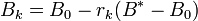Since Bn = 0 we can solve for B*,Substituting back into the formula for the Bk shows that they are a linear function of the rk and therefore the λk,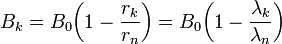This is the easiest way of estimating the balances if the λk are known. Substituting into the first formula for Bk above and solving for λk+1 we get,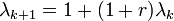λ0 and λn can be found using the formula for λk above or computing the λk recursively from λ0 = 0 to λn.

Since p=rB* the formula for the payment reduces to,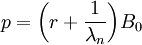and the average interest rate over the period of the loan is,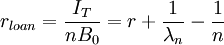which is less than r if n>1.

Look up interest in Wiktionary, the free dictionary.

## References

### Specific references

1. ^ ^ Johnson, Paul: A History of the Jews (New York: HarperCollins Publishers, 1987) ISBN 0-06-091533-1. p.174
2. ^ ^ 15 U.S.C. § 1615

### General references

• Duffie, Darrell and Kenneth J. Singleton (2003). Credit Risk: Pricing, Measurement, and Management. Princeton University Press. ISBN13 978-0691090467.
• Kellison, Stephen G. (1970). The Theory of Interest. Richard D. Irwin, Inc.. Library of Congress Catalog Card No. 79-98251.
• Lando, David (2004). Credit Risk Modeling: Theory and Applications. Princeton University Press. ISBN13 978-0691089294.
• van Deventer, Donald R. and Kenji Imai (2003). Credit Risk Models and the Basel Accords. John Wiley & Sons. ISBN13 978-0470820919.